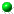Interactions of cultures and top people of Wikipedia from ranking of 24 language editions
by Y.-H.Eom, P.Aragon, D.Laniado, A.Kaltenbrunner, S.Vigna and D.L.Shepelyansky
arXiv:1405.7183[cs.SI]World map of top historical figures of 24 Wikipedia editions from PageRank
(darkness is proportional to number of appearances)

 ` `Article download: v1 (here); support information v1 (here); v2 (here), sup info v2 (here)Article published PLoS ONE 10(3): e0114825 (2015) with SI (here)Related Wikipedia article "Top 100 historical figures of Wikipedia" (here)World distribution of global top PageRank people by number of appearances "a" (here), by number of persons "p" (here); by number of persons in AD 15th to BC 5th centuries (here), BC 6th to BC 15th centuries (here), BC 16th to BC 20th centuries (here) [requires recent browser versions]World distribution of global top 2DRank people by number of appearances "a" (here), by number of persons "p" (here); by number of persons in AD 15th to BC 5th centuries (here), BC 6th to BC 15th centuries (here), BC 16th to BC 20th centuries (here) [requires recent browser versions]Above World distributions in tif gziped files (2.9 Mb) (here)Top 100 people from Hart's list: (here); from Pantheon MIT project: (here); overlap of Wikis with Hart's list (here)Global list of Wiki persons: in PageRank - 1045 names - (here), in 2DRank - 1616 names - (here)Global list of female Wiki persons: in PageRank (here), in 2DRank (here)List of country codes (here)Top 100 Wiki persons in PageRank: AR (here), DA (here), DE (here), EL (here), EN (here), ES (here), FA (here), FR (here), HE (here), HI (here), HU (here), IT (here), JA (here), KO (here), MS (here), NL (here), PL (here), PT (here), RU (here), SV (here), TH (here), TR (here), VI (here), ZH (here)Top 100 Wiki persons in 2DRank: AR (here), DA (here), DE (here), EL (here), EN (here), ES (here), FA (here), FR (here), HE (here), HI (here), HU (here), IT (here), JA (here), KO (here), MS (here), NL (here), PL (here), PT (here), RU (here), SV (here), TH (here), TR (here), VI (here), ZH (here)Above listings with tabulator divided data (here)Top 10 local Wiki persons from global PageRank and 2DRank lists for their own languages: AR (here), DA (here), DE (here), EL (here), EN (here), ES (here), FA (here), FR (here), HE (here), HI (here), HU (here), IT (here), JA (here), KO (here), MS (here), NL (here), PL (here), PT (here), RU (here), SV (here), TH (here), TR (here), VI (here), ZH (here), WR (world) (here)Datasets of 24 networks of 24 Wikipedia editions (2.4 Gb) (here), data have been generated by Sebastiano Vigna (http://vigna.di.unimi.it/)All 24wiki articles in order of PageRank (216Mb) (here), 2DRank (216Mb) (here) This webpage is created at May 6, 2014 and is maintained by D.L.Shepelyansky